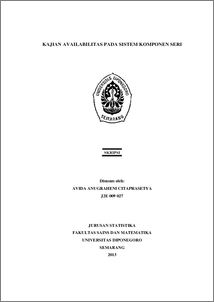# KAJIAN AVAILABILITAS PADA SISTEM KOMPONEN SERI

AVIDA, AVIDA (2013) KAJIAN AVAILABILITAS PADA SISTEM KOMPONEN SERI. Undergraduate thesis, UNIVERSITAS DIPONEGORO.Preview
PDF
556Kb

## Abstract

Availability is a measure of item or system performance. Availability of series system is derived from inherent availability of item that takes effect from mean time to failure (MTTF) and mean time to repair (MTTR). Given observed time data of microcontroller consists of processor core, memory and programmable I/O peripheral in series, is measured its system availability. By simple linier regression method, the parameter estimation is determined for the mean time after data distribution known. Processor core has Weibull distribution for failure time data with β ̂=1.17, η ̂=12000 and (y_i ) ̂=-11.0142+1.1726x_i as regression model while repair time data is lognormal distribution with (μ_l ) ̂=2.83, (σ_l ) ̂=1.34 and regression model is (y_i ) ̂=-2.1078+0.8322x_i. Memory has exponential failure time data with λ ̂=0.00005 and (y_i ) ̂=0.00005x_i as regression model while normal repair time data has μ ̂=27 dan σ ̂=14.49 and regression model is (y_i ) ̂=-1.8632+0.0699x_i. Failure time data distribution of programmable I/O peripherals is Weibull with β ̂=1.21, η ̂=20000 and regression model (y_i ) ̂=-11.9695+1.2086x_i while lognormal repair time data has (μ_l ) ̂=3.05, (σ_l ) ̂=1.1 and regression model is (y_i ) ̂=-2.7677+0.9069x_i. Due to MTTF is 11364.57 hours and MTTR is 41.59 hours, processor core’s availability is 99.64%. Availability of memory is 99.87% from MTTF is 20000 hours and MTTR is 27 hours. Programmable I/O peripheral has 18773.41 hours as MTTF and MTTR is 38.67 hours that deliver availability 99.79%. The series system availability is 99.30% means the probability of system is in the state of functioning at given time is 99.30%. Keywords: Availability, MTTF, MTTR, Regression.

Item Type: Thesis (Undergraduate) H Social Sciences > HA Statistics Faculty of Science and Mathematics > Department of Statistics 42345 Mr Hasbi Yasin 20 Feb 2014 09:38 04 Mar 2014 11:25

Repository Staff Only: item control page